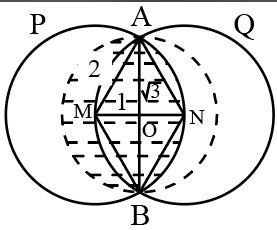Question 26

# Two circles P and Q, each of radius 2 cm, pass through each other’s centres. They intersect at points A and B. A circle R is drawn with diameter AB. What is the area of overlap (in square cm) between the circles R and P?

SolutionWe know that radius of circle P is 2 cm

Length of MO = 1 cm

radius of circle R = AO = $$\sqrt{\ 2^2-1^2}$$ = $$\sqrt{\ 3}$$

Area of overlap between the circle R and P(shaded region) = semi circle area of R + area of segment ANBOA

Area of segment ANBOA = area of sector ANBM - area of triangle AMB

= $$\frac{120}{360}\pi\ \left(2\right)^2$$ - $$\frac{1}{2}\left(1\right)\left(2\sqrt{\ 3}\right)$$

= $$\frac{4\pi}{3}-\sqrt{\ 3}$$

Area of overlap between circle R and P = $$\frac{\pi\ \left(\sqrt{\ 3}\right)^2}{2}$$+ $$\frac{4\pi}{3}-\sqrt{\ 3}$$

= $$\ \frac{\ 17\pi\ }{6}-\sqrt{\ 3}$$

• All Quant Formulas and shortcuts PDF
• 40+ previous papers with solutions PDF